International
Tables for
Crystallography
Volume D
Physical properties of crystals
Edited by A. Authier

International Tables for Crystallography (2006). Vol. D, ch. 1.1, p. 10

## Section 1.1.3.8. Tensor derivatives

A. Authiera*

aInstitut de Minéralogie et de la Physique des Milieux Condensés, Bâtiment 7, 140 rue de Lourmel, 75015 Paris, France
Correspondence e-mail: aauthier@wanadoo.fr

| top | pdf |

#### 1.1.3.8.1. Interpretation of the coefficients of the matrix – change of coordinates

| top | pdf |

We have under a change of axes: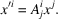This shows that the new components,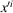, can be considered linear functions of the old components,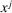, and one can write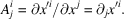It should be noted that the covariance has been increased.

#### 1.1.3.8.2. Generalization

| top | pdf |

Consider a field of tensors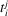that are functions of space variables. In a change of coordinate system, one has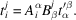Differentiate with respect to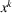: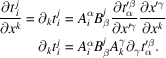It can be seen that the partial derivatives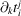behave under a change of axes like a tensor of rank 3 whose covariance has been increased by 1 with respect to that of the tensor. It is therefore possible to introduce a tensor of rank 1,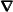(nabla), of which the components are the operators given by the partial derivatives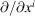.

#### 1.1.3.8.3. Differential operators

| top | pdf |

If one applies the operator nabla to a scalar ϕ, one obtains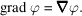This is a covariant vector in reciprocal space.

Now let us form the tensor product ofby a vector v of variable components. We then have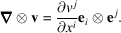The quantities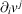form a tensor of rank 2. If we contract it, we obtain the divergence of v: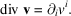Taking the vector product, we get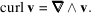The curl is then an axial vector.

#### 1.1.3.8.4. Development of a vector function in a Taylor series

| top | pdf |

Let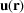be a vector function. Its development as a Taylor series is written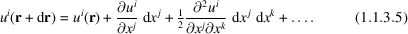The coefficients of the expansion,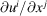,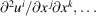are tensors of rank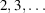.

An example is given by the relation between displacement and electric field: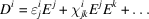(see Sections 1.6.2and 1.7.2).

We see that the linear relation usually employed is in reality a development that is arrested at the first term. The second term corresponds to nonlinear optics. In general, it is very small but is not negligible in ferroelectric crystals in the neighbourhood of the ferroelectric–paraelectric transition. Nonlinear optics are studied in Chapter 1.7.